# How to paste opposite in Excel- 3 easy ways

Copying and pasting is a normal occurrence in Excel. Most times, we are used to copying data and pasting it as it is. But what if you need to copy and paste a list of column or row data in reverse order? There is no direct in-built function in Excel that reverses a list of Excel data. Despite this, it is not a hard task to achieve as Excel offers users different tools that can enable them to paste in reverse.

The article below discusses how to paste in reverse order in Excel in a few simple steps.

## Method 1: flipping a column in Excel

You can copy and paste in reverse using the descending feature in Excel. Here we explain how you can reverse the order of data in a column vertically with simple steps:

1. In an open Excel workbook, add a helper column next to the column you want to reverse.

2. Populate the helper column with a sequence of numbers, starting with 1.

3. Drag the AutoFill handle to continue numbering the columns in descending order.4. Select the helper column and select the Data tab on the main menu ribbon.

5. In the Sort & Filter group, click on the icon representing sorting from Largest to Smallest (ZA).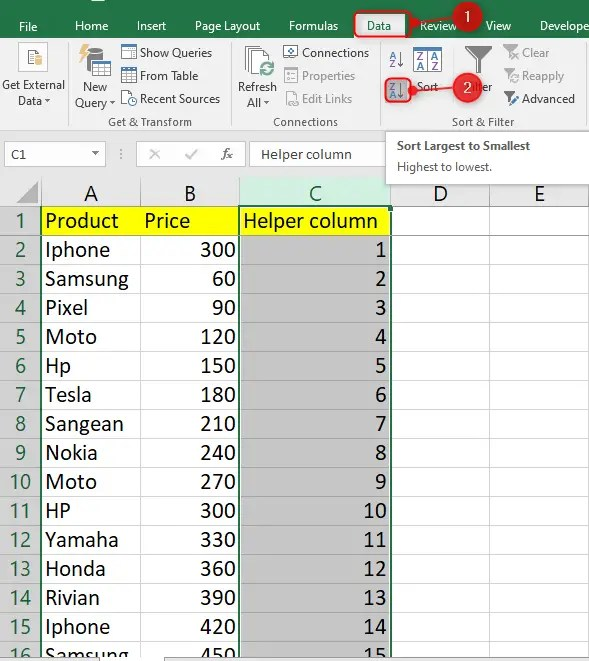A sort prompt warning will pop up, choose to expand the selection to rearrange all of the data

You will notice that the helper column is arranged to reverse the order, and the main column data is also in reverse order.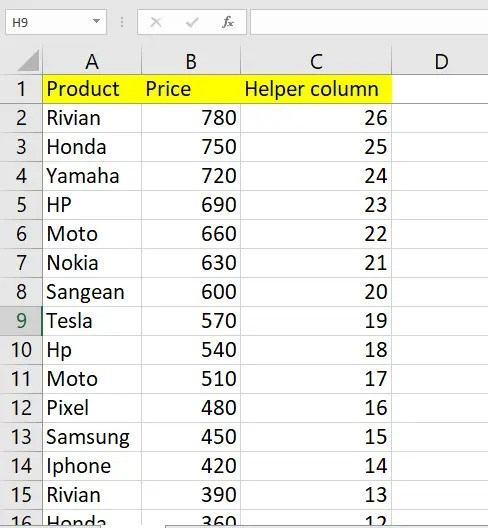## Method 2: Copy and paste in reverse order with a Formula

You can also apply a formula to reverse a list of column cells. Enter the following formula into a blank cell where you want to reverse the column order:

=OFFSET (last cell,-(ROW (first cell)-1, 0)

For instance, if your first cell with data is B1 and the last cell is A15, your formula will be:

=OFFSET(\$B\$15,-(ROW(B1)-1),0)

First copy and paste as usual then follow the steps below to turn the contents opposite

Write the formula in a blank cell and press enter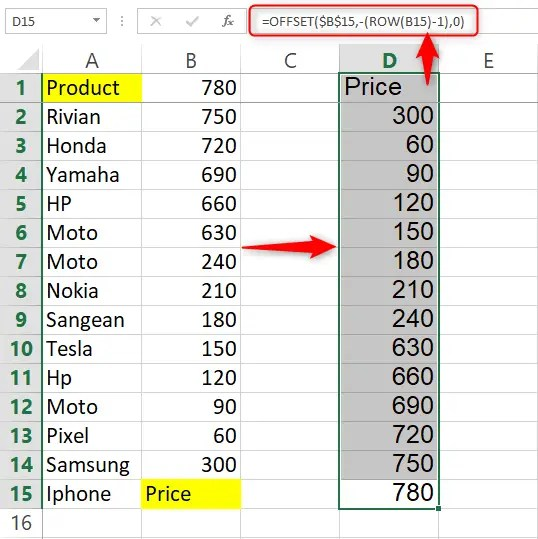As you can see from the image above the offset function has rea arranged the data in reverse order. The contents that were at the bottom are now on top. You can delete the helper columns after turning the data in reverse order. Just highlight the columns by clicking their respective letters then hit the delete button.

## Method 3: Copy and paste in reverse order horizontally

You can copy and paste a list of rows in reverse order with a formula. Here, we give you the formula to use when pasting row values in reverse order.

In an empty cell, copy the formula below

=OFFSET (first cell, COUNTA (row number)-COLUMN (first cell),)

You can apply the formula to the cells below by dragging the AutoFill handle.

=OFFSET(\$A\$1,,COUNTA(1:1)-COLUMN(A1),)

Enter the above formula in a blank cell then click on enter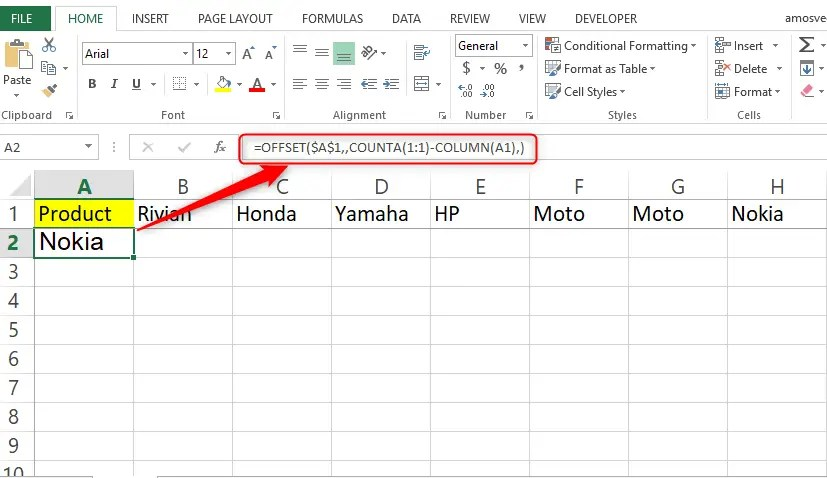Drag the formula to reverse the remaining cells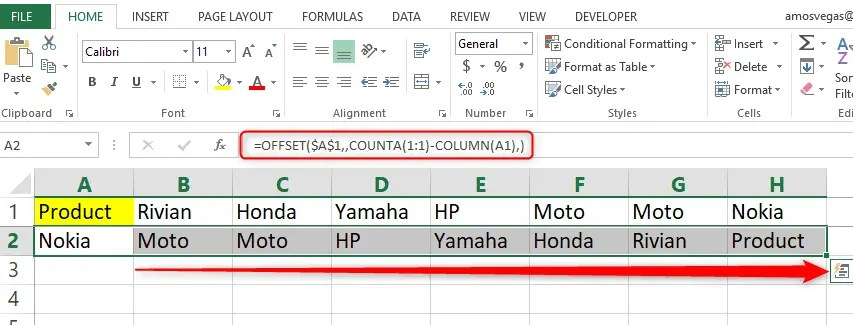That’s how to copy and paste in opposite direction in Excel. If you are looking to paste in a row rather than a column and vice versa, then you should choose transpose in the paste option.  Remember using the formulas to post the opposite, it only posts the values only.